Impact Factor 2.705 | CiteScore 2.2
More on impact ›

# Frontiers in Materials## Original Research ARTICLE

Front. Mater., 03 June 2020 | https://doi.org/10.3389/fmats.2020.00163

# Phase Field Modeling of Dendritic Growth on Spherical SurfacesLaureano Ortellado andLeopoldo R. Gómez*
• Department of Physics, Universidad Nacional del Sur—IFISUR—CONICET, Bahía Blanca, Argentina

The spontaneous formation of a crystal phase is one of the most common and beautiful pattern formation mechanisms in nature. Different instabilities in the crystal interface may lead to the growth of ramified structures, known as dendritic crystal growth. In this work, we use a Phase Field Model and numerical simulations to study 2D dendritic growth on curved surfaces. We show how the degree of ramification of a growing nucleus is modified by the underlying curvature of the substrate.

## 1. Introduction

The formation of a crystalline structure from an initial liquid phase (crystallization), is one of the richest pattern formation processes, with deep consequences in condensed matter physics and chemistry, material science, and even biology (Kashchiev, 2000; Kelton and Greer, 2010). For years this process has been scrutinized by means of experiments, simulations, and mathematical models, in both 3D and 2D systems.

In general, the fundamental mechanism of crystallization is given by nucleation and growth, where an initial fluctuation in the liquid spontaneously forms a small seed of the equilibrium crystal. In the simplest picture, there are only two energies associated with this seed (Kashchiev, 2000; Kelton and Greer, 2010). There is an energy gain due to the formation of a piece of the equilibrium (less energetic) structure. But there is also an energy penalty due to the formation of an interface between the crystal and the liquid. This competition leads to an activated process where only those seeds overcoming a critical size are able to grow, while the rest collapse and disappear by surface tension.

Conventional nucleation and growth commonly leads to the formation of growing compact nuclei, with typical polygonal shapes depending on the anisotropies of the surface tension. However, instabilities in the liquid-crystal interface, originated for example by the diffusion of heat needed in the formation of the crystal phase, may lead to the ramifications of the nuclei, in a process known as dendritic crystal growth (Langer, 1980).

On the other side, in the last years, condensed matter scientists have also begun to study the properties of 2D ordered phases deposited on curved substrates (Nelson, 2002; Vitelli and Nelson, 2004; Vitelli et al., 2006; Irvine et al., 2010; Tarjus et al., 2012; Garćıa et al., 2013, 2015; Gómez et al., 2018). In such cases, it has been shown that the underlying curvature of the substrates modifies the structure of the phases, such that topological defects, like dislocations and disclinations, equilibrate and locate on particular regions of the substrate. This is because, on curved geometries, topological defects may help to reduce the strain energy in the ordered phases (crystal or liquid crystal phases).

In the same line, it was recently shown that the curvature of substrates can also affect the crystallization dynamics and mechanism of crystal growth on curved surfaces (Meng et al., 2014; Horsley et al., 2018). This is because curvature modifies the imbalance of volume and surface free energies of nuclei leading to crystallization. For example, in the case of nucleation on spherical substrates, it was shown that the size of a critical nucleus Rc on a sphere of radius a decreases, as compared with the critical size (at same conditions) on the plane ${R}_{c}^{0}$, following the expression (Gómez et al., 2015):

Thus, it is easier to nucleate on a sphere than on a plane. This is just because on a sphere a nucleus has a bigger ratio between volume and surface energy, favoring the nucleation of the equilibrium phase. Such expression, obtained from a coarse grained phase field model, has been confirmed by molecular dynamic simulations (Law et al., 2018), and complementary theoretical calculations (Horsley et al., 2018).

On curved surfaces, it was also found that curvature modifies the shape of critical and growing nuclei (Meng et al., 2014; Köhler et al., 2015, 2016; Paquay et al., 2017; Ma et al., 2019,?; Wang et al., 2019). This is mainly observed when the growing nuclei are unable (due to the particles' interactions) to relax the strain energy by nucleating topological defects (dislocations and disclinations). In such cases, curvature induces and elastic instability which produces the growth of ramified un-defected nuclei.

There is also an interest in understanding the features of irregular crystal growth on curved surfaces. In this sense, the dendrite growth of metal patches on colloidal particles was recently experimentally studied (Bihr et al., 2017), and it was also experimentally showed that the mechanism of freezing of soap bubbles (crystallization on spheres) may involve convection flows transporting seeds and small crystallites across the system (Ahmadi et al., 2019). In addition to that, crystal growth on the non-plane surface has proven to be relevant in understanding the structures of virus (Ganser et al., 1999; Dharmavaram et al., 2017)

Here, we use numerical simulations to study the features of adiabatic crystal growth on curved surfaces. For the sake of concreteness, and due to the experimental relevance, here we will focus on crystal growth on spherical substrates. By using simple models, we will show how the curvature of spheres modifies the growth of compact and dendritic (ramified) nuclei.

## 2. Model

Here we study crystallization in curved geometries within the framework of the Phase Field Model approach (Provatas and Elder, 2011), by using a scalar and real order parameter ϕ(r, t). Points on the surface of the substrate are specified by curvilinear coordinates r = (x1, x2). In this coordinates, the metric of the surface takes the form $d{s}^{2}={g}_{\alpha \beta }d{x}^{\alpha }d{x}^{\beta }$, where gαβ is the metric tensor (O'Neill, 1997). The order parameter ϕ is a measure of the degree of order in the system during the phase transition, its values ranged from 0 when the system is in the disorder (liquid) phase, to 1 when the ordered (crystal) phase has been formed.

In this model, the phase equilibria is described in terms of the total free energy F of the system expanded as a functional of ϕ(r, t). In general, the free energy for a mixed state system is express as (Yu et al., 2017):

The term e(r) represents the magnitude of penalization in the free energy by the interphase, and f(ϕ, T) is the local free energy density of a homogeneous system having an order parameter ϕ at temperature T, and g is the determinant of the metric tensor. The dimensionless temperature T is such that T = 0 is the subcooling temperature, and Te = 1 is the two-phase equilibrium temperature. The function f has the typical shape of a double well potential with two local minima, one of them ϕ = 0 corresponding to the liquid, and the other in ϕ = 1 to the crystal phase. The competition between the desire of the system to remain in one of the bulk phase minima of f (liquid or solid) and the cost of high gradients results in a finite interface width (Warren et al., 2003). The local free energy f is represented as:

where the parameter m(T) controls the driving force to the crystal phase and w controls the height of the double well. Here we have set w = 1.

In the specific case of a liquid-solid interphase, the parameter e(r) represents surface tension of the crystal that depends on the direction of the crystal orientation. In curvilinear coordinates, the crystal orientation is measured by the angle ψ that the crystal makes with the $\stackrel{^}{{x}_{1}}$ versor, i.e., $tan\left(\psi \right)=\left(\frac{-{\partial }_{{x}_{2}}\varphi /\sqrt{{g}_{22}}}{-{\partial }_{{x}_{1}}\varphi /\sqrt{{g}_{11}}}\right)$. Then

where e0 controls the magnitude of penalization in the free energy, n represents the anisotropy of the system (n = 4 for a square, n = 6 for a hexagon, and so on), and δ0 controls the strength of the anisotropy.

In this approach the dynamics of the phase transition can be studied through a relaxational equation of the form (at constant T):

where μϕ is the mobility of the system and $\frac{\delta F}{\delta \varphi }$ is the functional derivative of F in terms of the order parameter ϕ. Defining the free energy F as F = ∫L(ϕ, ▽ϕ, T)d2r, the functional derivative is written:

where the curvilinear gradient and divergence are $▽={g}^{\alpha \beta }{\partial }_{\alpha }$ and $▽·=\frac{1}{\sqrt{g}}{\partial }_{\alpha }\sqrt{g}$.

In the case of adiabatic crystal growth, the effects of temperature variation in the neighbor of the liquid/crystal interface are key, and an equation for the diffusion of heat is also needed. Note that local changes in the temperature modifies the parameter m(T), which controls the deep of the double well [$\Delta f=f\left(0,m\right)-f\left(1,m\right)=\frac{m}{6}$]. An evolution equation for the temperature field can be obtained from the conservation of enthalpy:

where ΔLB represents the Laplace-Beltrami operator ${\Delta }_{LB}=\frac{1}{\sqrt{g}}{\partial }_{\alpha }\sqrt{g}\left({g}^{\alpha \beta }{\partial }_{\beta }\right)$. Here K is an adimensional parameter, proportional to the latent heat and inversely proportional to the heat capacity of the solid phase. For the sake of simplicity, we establish the same constant of diffusion for the solid and liquid phases.

Since the driving force of the interphase is the subcooling, the parameter m must be a function of m(TeT). In that way, we can model m as:

where K1 and K2 are parameters such that K1 < 1 thus $|m|<\frac{1}{2}$.

Thus, in this model the adiabatic evolution of the system is given by the coupled differential Equations (5) and (7). To study crystal growth on the sphere we write the equations in spherical coordinates, where points on the sphere are specified by r = (θ, φ) where x1 = θ ∈ [0, π] is the polar angle measured from a fixed zenith direction, and x2 = φ ∈ [0, 2π) is the azimuth angle, Figure 1 shows the mesh on the sphere due to the spherical parametrization. In these coordinates, the arc length ds is given by ds2 ≡ |dr|2 = a22 + sin(θ)2a22, and the differential operators take the form:

FIGURE 1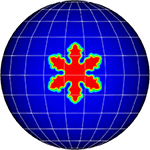Figure 1. Scheme of the computational approach used to study dendritic growth on spherical substrates. The evolution equations are solved in spherical coordinates. The number of grid-points are adjusted to obtain equivalent grids on substrates of different curvature.

The evolution equations are numerically solved by a finite difference scheme, forward in time and centered in space. As the initial crystal seed we use a geodesic circle. Multiple growing nuclei can be modeled by seeding different circular (geodesic) seeds on different parts of the sphere.

Typically, we set the parameters e0 = 0.01, ${\mu }_{\varphi }=\frac{1}{0.0003}$, δ0 = 0.01 . To model the double potential well we set K1 = 0.9 and K2 = 10. Due to the spherical coordinates, our computational grid is not uniform, see an scheme in Figure 1, and the cell size decreases in the polar angle direction. At the pole of the sphere the element size is zero, and hence, the pole is singular point. Therefore, in our analysis, we ignore the growth near the poles. In order to use equivalent grids, we vary the number of grid points for spherical substrates of different radius (up to nθ × nφ = 512 × 1024 for a sphere of radius 4). The angular step, are then, $\Delta \theta =\frac{\pi }{{n}_{\theta }}$ and $\Delta \phi =\frac{2\pi }{{n}_{\phi }}$.

## 3. Results

In order to test the model, we first consider isothermal crystallization on spheres. Figure 2 shows snapshots at different times of isothermal growth of nuclei for different degrees of anisotropy. From top to bottom the different rows in this figure show the evolution of the system for n = 1, n = 3, n = 4, and n = 6, respectively. As clearly seen from the panels, on spheres, initial circular nuclei acquire symmetric shapes as a consequence of the symmetry in the surface tension, similarly to isothermal crystallization in planar geometries (Kashchiev, 2000; Kelton and Greer, 2010).

FIGURE 2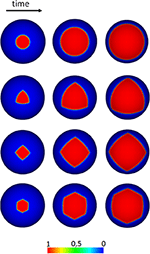Figure 2. Isothermal crystallization on spheres. This figure shows the isothermal growth of initial circular nuclei on spheres (time flows from left to right), for different surface tension anisotropy. From top to bottom the different rows show the evolution of nuclei for anisotropies n = 1, n = 3, n = 4, and n = 6. At the bottom we show the color map of the order parameter ϕ.

We now consider the features of dendritic growth on spherical substrates. Figure 3 shows the shape of dendritic nuclei grown on spherical substrates of different radius a = 1.5, 2, 3, 4. To make this comparison we use the same circular seed of geodesic radius r = 0.25, and the panels show the crystallites at the same time t = 0.1.

FIGURE 3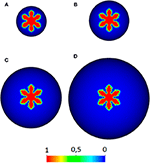Figure 3. Shape of dendrites as a function of curvature. This figure compares the shape of dendrites grown on substrates of different curvature. Panels (A–D) correspond to crystallites grown on substrates of radius a = 1.5, 2, 3, 4, respectively. Note that for small substrates (higher curvature) the dendrites look less ramified. The color bar shows the amplitude of the order parameter ϕ.

From this figure it can be qualitatively observed that the nuclei look less ramified on substrates of smaller size (compare Figures 3A,D). This suggests that curvature may originate a reduction in the ramifications of nuclei. In order to study this in more detail we calculate the degree of Circularity C of nuclei under same conditions, but on spherical substrates of different radius:

where A is the area of the nucleus, and L its perimeter. Note that the Circularity of a planar circular nucleus is C ≡ 1, while the Circularity of a fractal nuclei approaches zero C ≡ 0.

Figure 4 shows the Circularity of nuclei grown on different spherical substrates. Note that for different values of K, the circularity C continuously increases for smaller substrates, showing that the curvature contributes to the reduction of ramifications and the propagation of more compact nuclei. Phase field models can be sensitive to the discretization (mesh-induced anisotropy). In order to study if these results are influenced by the meshes, we have run simulations of growing crystal of different orientations (Dobravec et al., 2020). The variations observed in the circularity plotted as error bars in Figure 4, and are of the order of symbol size showing the robustness of the results.

FIGURE 4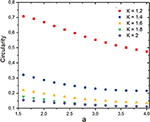Figure 4. Circularity of dendrites. This figure shows the Circularity of dendrites at time t = 0.1 for nuclei grown on substrates of different radii a, and for different values of the constant K (error bars are of the order of symbol size and omitted for clarity). Note that for smaller (higher curvature) the nuclei become less ramified.

It is also interesting to study how the substrate's curvature influences the selection of tip radius and propagation velocity of dendrites. In order to obtain the tip radius, we measured from the simulations the geodesic curvature of the interface at the tips as a function of the size of the spherical substrate. Suppose that the curvature of a curve C belonging to a surface S is k. The curvature of the curve at that point can be composed in two components, the normal curvature kn and the geodesic curvature kg (see Figure 5) (O'Neill, 1997). While the normal curvature is obtained by projecting curvature vector on the surface normal, the geodesic curvature is obtained by projecting on the tangential plane at that point. The curvatures are related by the identity:

FIGURE 5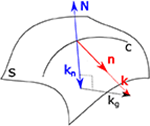Figure 5. Sketch of the curvature of curve embedded in a surface in the 3D-space. This figure shows the curvature k on curve C which has a normal n on a surface S. The normal of the surface is N, and its projection with the curvature k is the normal curvature kn. The geodesic curvature kg, that is perpendicular to kn is, by definition, ${k}_{g}^{2}={k}^{2}-{k}_{n}^{2}$.

Note that the geodesic curvature is the intrinsic curvature of a curve inside the surface, and its the relevant quantity related to curvature-driven growth on surfaces. To measure the tip radii from the simulations, we first extracted the contour interface and calculated the curvature k at the tip by using the Frenet-Serret formulas. We then calculate the normal curvature kn by projecting to the sphere's normal and that point. Then, the geodesic curvature of the tip is obtained from Equation (13). Finally, the radius of curvature Rg of the tip can be approximated by ${k}_{g}\approx \frac{1}{{R}_{g}}$, which is valid for small circles on a sphere, as shown in Figure 6.

FIGURE 6Figure 6. Dendrite's tip. (A) Phase-field simulation of a dendrite (sphere of radius 3 and time t = 0.1). (B) Interface contour. (C) Approximated tip radius R.

Figure 7 shows how surface curvature affects the selection criterion. Here we plot the selected velocity of the tips as a function of the radius of the tip, for dendritic crystals growing on spheres of different curvature (the radius of the spherical substrates is indicated right on the side of the data). Note that as the size of the spherical substrate decreases, the selected tip radius increases. This is the same as reported above, the dendrites get more rounded for spherical substrates of higher curvature (smaller spheres). On the contrary, the selected velocity shows a non-monotonic behavior. For smaller spherical substrates the tip velocity increases at first, but later starts to decrease. It is interesting to note that a similar non-monotonic behavior of the tip velocity as a function of tip radius is also observed in planar geometries when a different degree of undercooling are used. Such behavior is roughly described by the spherical approximation which considers the tip of the dendrite as a growing sphere (Langer, 1980). Our simulations show that not only the undercooling, but also the substrate's curvature selects both, tip's radius and velocity.

FIGURE 7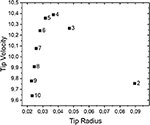Figure 7. Tip velocity as a function of the tip radius. This figure shows the tip velocity as function of the tip radius for dendrites growing on spheres of different radii (labeled at the right of each symbol). Errors bar are of the order of symbol size and omitted for clarity.

In order to rationalize how the underlying curvature can modify the shape of dendrites on curved substrates, here we consider the interfacial instabilities associated with dendritic growth. To do this we use an equation describing the temporal evolution of the interface curvature:

where , and s and κg are the arc length and the geodesic (intrinsic) curvature of the interface. Originally, this equation was used as a geometrical model for interface evolution in a variety of different problems (Brower et al., 1984; Kessler et al., 1984), including dendritic crystallization. Although in the original model the key property of the interface was the total curvature κ, here we slightly modify the model by using the geodesic curvature κg, which is the curvature of the interface as seen from the curved substrate. In general, the geodesic curvature of a curve is also known as the intrinsic curvature of a curve (O'Neill, 1997).

We now use this model to develop an instability analysis of initial circular nuclei growing on a spherical substrate. This can be done by considering normal perturbations to an initial circular nuclei of geodesic radius r, such that the equation of the interface can be written as $\stackrel{⃗}{x}\left(\phi ,t\right)=\left[r\left(t\right)+ϵ\left(\phi ,t\right)\right]$, where ϵ is the perturbation and φ is the azimuthal angle.

In general, the geodesic curvature of a closed curve on the sphere can be written as (Parisio et al., 2001):

where r is the local geodesic radius of the curve (the coordinates r, φ are usually known as geodesic polar coordinates; Gómez et al., 2015).

Thus, to first order in the perturbation ϵ, we can approximate:

Now, by expanding the perturbation in the polar angle using the eigenfunctions ${ϵ}_{m}={ϵ}_{0}{e}^{{\lambda }_{m}t}cos\left(m\phi \right)$, we obtain the growth rate:

We note that for large spherical substrates (r < < a) the above expression reduces to growth rate obtained previously for dendritic growth in the plane ${\lambda }_{m}^{0}=\left({m}^{2}-1\right)\left[{V}^{\prime }\left(1/r\right)-\gamma {m}^{2}/{r}^{2}\right]/{r}^{2}$ (Brower et al., 1984; Kessler et al., 1984).

Thus, the first correction of the amplification factor due to curvature can be written as ${\lambda }_{m}\approx {\lambda }_{m}^{0}/\left(1-{r}^{2}/3{a}^{2}\right)$, such that although the growing nuclei are still linearly unstable on spheres, the exponential amplification of perturbations reduces for higher curvatures.

In order to study the evolution of the interface at early times (linear behavior), but also at long times (non-linear behavior), we numerically solve Equation (14), on spherical substrates of different sizes, by using a predictor-corrector approach. Figure 8A shows a typical interface morphology obtained at early times, as seen in the θ − φ space (t = 0.116). In Figure 8B we show that anisotropic dendritic growth on spheres can also be modeled by including a factor 1 + εcos(), multiplying the right hand of equation 14 (this figure corresponds to a simulation with hexagonal symmetry n = 6). In the lower panel of Figure 8C, we show the interface deviation from a geodesic circle ϵ, as a function of φ (t = 0.056). This can be used to analyze the evolution of the different modes during dendritic growth. In the top panels of Figure 8C we show the structure factor of the lower figure, and the evolution of the peak of the structure factor. As predicted by the linear analysis there is a range of unstable modes, and the amplification factor grows exponentially in time, until the non-linearities of the equation slows-down the growth, similarly as occur in other unstable systems like spinodal decomposition (Vega and Gómez, 2009).

FIGURE 8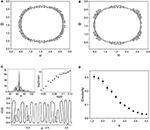Figure 8. Interface analysis of crystal growth. This figure shows the analysis of the interface of a growing nuclei. Panels (A,B) correspond to the crystal interface of isotropic and six-fold symmetry, respectively, as seen in the θ − φ plane (t = 0.116). Panel (C) shows the crystal interface deviation form a geodesic circle ϵ(φ) at t = 0.056 (below), the structure factor of this interface configuration (upper left), and the exponential growth of the peak of the structure factor (upper right). Panel (D) shows the circularity of the growing nuclei for a spherical substrate of different radius a, as obtained by the numerical solution of the interface model (t = 0.0001, and α = β = γ = 1).

It is worth to study the degree of ramifications as obtained during dendritic growth for this interface equation model. In Figure 8D, we show the circularity of growing nuclei, for spherical substrates of different sizes. Similarly to obtained with the phase field model, here we also observe a reduction of ramifications as the size of the substrate is reduced. Here, we note that a similar result was obtained for a phase field crystal model, where the degree of ramifications of growing nuclei was also observed to be reduced for smaller spheres. In such cases it was observed that growing nuclei evolve from a sixfold branching geometry to a ribbon as the size of the spherical surface is reduced (Köhler et al., 2016). Note that our results show that the reduction of ramification by curvature is also found even when the elastic interaction terms due to the crystal lattice are not taken into account, as in our model.

A similar mode expansion analysis was developed to study the instability of an interface separating two immiscible viscous fluids on a sphere (the Saffman-Taylor problem on a sphere) (Parisio et al., 2001). In such a work, a perturbation analysis up to second order in ϵ, showed an asymmetry on the tip-splitting behavior depending on the region where the interface was evolving. While tip-splitting is still present for interfaces on the northern hemisphere, tip-splitting completely vanishes in the southern hemisphere, where is completely replaced by a finger tip-sharpening mechanism.

Here we also found that tip-splitting is suppressed for large nuclei whose interface goes beyond the equator. Figure 9 shows the temporal evolution of a large crystal (in red). Note that the interface still becomes linearly unstable, but there is no tip-splitting leading to dendritic patterns. As can be observed from panel Figures 9B,C there is a tip-narrowing mechanism rather than tip splitting, similarly as predicted for fluids (Parisio et al., 2001). This is a simple direct consequence of the geometry and topology of the sphere, where such a large growing nuclei produce a converging flow, where the mechanism of tip-splitting is completely suppressed (Thomé et al., 1989).

FIGURE 9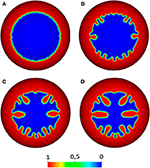Figure 9. Temporal evolution of a large crystal seed. This figure shows the temporal evolution of a large crystal seed of geodesic radius of r/a = 4.78 on a sphere of radius a = 2. Panels (A–D) correspond to t = 0.26, 0.11, 0.18, 0.26, respectively. Note that tip-splitting is replaced by tip narrowing. The color bar shows the amplitude of the order parameter ϕ.

## 4. Conclusions

In this work, we have explored the features of dendritic crystal growth on spherical substrates. In general, the underlying curvature of the substrates can be used to control the shape of crystals and propagation velocity. We found a reduction in the ramification of growing nuclei as a consequence of the curvature of spheres, which can be understood from a linear instability analysis of the crystal-liquid interface. For large nuclei whose size surpass half the surface of the sphere (the interface goes beyond the equator), there is a vanishing tip-splitting mechanism. For such nuclei the interface is still unstable, but the non-linear process of tip-splitting is replaced by a tip-narrowing behavior. Our results show that geometry and topology can drastically affect crystallization and aggregation mechanisms on spherical substrates. This could help to control the features of crystalline textures on micro/nano particles.

## Data Availability Statement

The original contributions presented in the study are included in the article/supplementary materials, further inquiries can be directed to the corresponding author.

## Author Contributions

LG designed the research. LO performed the numerical simulations. Both authors discussed and analyzed the results and contributed to the manuscript preparation.

## Funding

This work was supported by the National Research Council of Argentina, CONICET, the Fondo para la Investigación Cientifica y Tecnologica (FONCYT, Grant PICT-2017-3611), and Universidad Nacional del Sur.

## Conflict of Interest

The authors declare that the research was conducted in the absence of any commercial or financial relationships that could be construed as a potential conflict of interest.

## Acknowledgments

We thank Daniel A. Vega and A. Juan for helpful discussions.

## References

Ahmadi, S. F., Nath, S., Kingett, C. M., Yue, P., and Boreyko, J. B. (2019). How soap bubbles freeze. Nat. Commun. 10:2531. doi: 10.1038/s41467-019-10021-6

Bihr, T., Sadafi, F.-Z., Seifert, U., Taylor, R. K., and Smith, A.-S. (2017). Radial growth in 2D revisited: the effect of finite density, binding affinity, reaction rates, and diffusion. Adv. Mater. Interfaces 4:1600310. doi: 10.1002/admi.201600310

Brower, R. C., Kessler, D. A., Koplik, J., and Levine, H. (1984). Geometrical models of interface evolution. Phys. Rev. A 29:1335. doi: 10.1103/PhysRevA.29.1335

Dharmavaram, S., Xie, F., Klug, W., Rudnick, J., and Bruinsma, R. (2017). Orientational phase transitions and the assembly of viral capsids. Phys. Rev. E 95:062402. doi: 10.1103/PhysRevE.95.062402

Dobravec, T., Mavrič, B., and Šarler, B. (2020). Reduction of discretisation-induced anisotropy in the phase-field modelling of dendritic growth by meshless approach. Comput. Mater. Sci. 172:109166. doi: 10.1016/j.commatsci.2019.109166

Ganser, B. K., Li, S., Klishko, V. Y., Finch, J. T., and Sundquist, W. I. (1999). Assembly and analysis of conical models for the HIV-1 core. Science 283, 80–83. doi: 10.1126/science.283.5398.80

García, N. A., Pezzutti, A. D., Register, R. A., Vega, D. A., and Gómez, L. R. (2015). Defect formation and coarsening in hexagonal 2D curved crystals. Soft Matter 11, 898–907. doi: 10.1039/C4SM02234C

García, N. A., Register, R. A., Vega, D. A., and Gómez, L. R. (2013). Crystallization dynamics on curved surfaces. Phys. Rev. E 88:012306. doi: 10.1103/PhysRevE.88.012306

Gómez, L. R., García, N. A., Vega, D. A., and Lorenzana, J. (2018). Thermal properties of vortices on curved surfaces. Phys. Rev. E 97:012117. doi: 10.1103/PhysRevE.97.012117

Gómez, L. R., García, N. A., Vitelli, V., Lorenzana, J., and Vega, D. A. (2015). Phase nucleation in curved space. Nat. Commun. 6:6856. doi: 10.1038/ncomms7856

Horsley, E. M., Lavrentovich, M. O., and Kamien, R. D. (2018). Aspects of nucleation on curved and flat surfaces. J. Chem. Phys. 148:234701. doi: 10.1063/1.5030752

Irvine, W. T., Vitelli, V., and Chaikin, P. M. (2010). Pleats in crystals on curved surfaces. Nature 468:947. doi: 10.1038/nature09620

Kashchiev, D. (2000). Nucleation. Oxford: Elsevier. doi: 10.1016/B978-075064682-6/50006-8

Kelton, K., and Greer, A. L. (2010). Nucleation in Condensed Matter: Applications in Materials and Biology, Vol. 15. Pergamon; Oxford: Elsevier.

Kessler, D. A., Koplik, J., and Levine, H. (1984). Geometrical models of interface evolution. II. numerical simulation. Phys. Rev. A 30:3161. doi: 10.1103/PhysRevA.30.3161

Köhler, C., Backofen, R., and Voigt, A. (2015). Relaxation of curvature-induced elastic stress by the asaro-tiller-grinfeld instability. Europhys. Lett. 111:48006. doi: 10.1209/0295-5075/111/48006

Köhler, C., Backofen, R., and Voigt, A. (2016). Stress induced branching of growing crystals on curved surfaces. Phys. Rev. Lett. 116:135502. doi: 10.1103/PhysRevLett.116.135502

Langer, J. S. (1980). Instabilities and pattern formation in crystal growth. Rev. Modern Phys. 52:1. doi: 10.1103/RevModPhys.52.1

Law, J. O., Wong, A. G., Kusumaatmaja, H., and Miller, M. A. (2018). Nucleation on a sphere: the roles of curvature, confinement and ensemble. Mol. Phys. 116, 3008–3019. doi: 10.1080/00268976.2018.1483041

Ma, L., Liu, X., Soh, A.-k., He, L., Wu, C., and Ni, Y. (2019). Growth of curved crystals: competition between topological defect nucleation and boundary branching. Soft Matter 15, 4391–4400. doi: 10.1039/C9SM00507B

Meng, G., Paulose, J., Nelson, D. R., and Manoharan, V. N. (2014). Elastic instability of a crystal growing on a curved surface. Science 343, 634–637. doi: 10.1126/science.1244827

Nelson, D. R. (2002). Defects and Geometry in Condensed Matter Physics. Cambridge: Cambridge University Press.

O'Neill, B. (1997). Elementary Differential Geometry. New York, NY: Academic Press.

Paquay, S., Both, G.-J., and van der Schoot, P. (2017). Impact of interaction range and curvature on crystal growth of particles confined to spherical surfaces. Phys. Rev. E 96:012611. doi: 10.1103/PhysRevE.96.012611

Parisio, F., Moraes, F., Miranda, J. A., and Widom, M. (2001). Saffman-Taylor problem on a sphere. Phys. Rev. E 63:036307. doi: 10.1103/PhysRevE.63.036307

Provatas, N., and Elder, K. (2011). Phase-Field Methods in Materials Science and Engineering. Weinheim: John Wiley & Sons. doi: 10.1002/9783527631520

Tarjus, G., Sausset, E., and Viot, P. (2012). Statistical mechanics of liquids and fluids in curved space. Adv. Chem. Phys. 148:251. doi: 10.1002/9781118158715.ch4

Thomé, H., Rabaud, M., Hakim, V., and Couder, Y. (1989). The Saffman-Taylor instability: from the linear to the circular geometry. Phys. Fluids A Fluid Dyn. 1, 224–240. doi: 10.1063/1.857493

Vega, D. A., and Gómez, L. R. (2009). Spinodal-assisted nucleation during symmetry-breaking phase transitions. Phys. Rev. E 79:051607. doi: 10.1103/PhysRevE.79.051607

Vitelli, V., Lucks, J. B., and Nelson, D. R. (2006). Crystallography on curved surfaces. Proc. Natl. Acad. Sci. U.S.A. 103, 12323–12328. doi: 10.1073/pnas.0602755103

Vitelli, V., and Nelson, D. R. (2004). Defect generation and deconfinement on corrugated topographies. Phys. Rev. E 70:051105. doi: 10.1103/PhysRevE.70.051105

Wang, K., Puretzky, A. A., Hu, Z., Srijanto, B. R., Li, X., Gupta, N., et al. (2019). Strain tolerance of two-dimensional crystal growth on curved surfaces. Sci. Adv. 5:eaav4028. doi: 10.1126/sciadv.aav4028

Warren, J. A., Kobayashi, R., Lobkovsky, A. E., and Carter, W. C. (2003). Extending phase field models of solidification to polycrystalline materials. Acta Mater. 51, 6035–6058. doi: 10.1016/S1359-6454(03)00388-4

Yu, H., Gupta, N., Hu, Z., Wang, K., Srijanto, B. R., Xiao, K., et al. (2017). Tilt grain boundary topology induced by substrate topography. ACS Nano 11, 8612–8618. doi: 10.1021/acsnano.7b03681

Keywords: dendritic growth, phase field, curvature, crystal interface, spherical substrate

Citation: Ortellado L and Gómez LR (2020) Phase Field Modeling of Dendritic Growth on Spherical Surfaces. Front. Mater. 7:163. doi: 10.3389/fmats.2020.00163

Received: 16 September 2019; Accepted: 04 May 2020;
Published: 03 June 2020.

Edited by:

P. Davide Cozzoli, University of Salento, Italy

Reviewed by:

Junseok Kim, Korea University, South Korea
Abhik Narayan Choudhury, Indian Institute of Science (IISc), India
Božidar Šarler, University of Ljubljana, Slovenia

Copyright © 2020 Ortellado and Gómez. This is an open-access article distributed under the terms of the Creative Commons Attribution License (CC BY). The use, distribution or reproduction in other forums is permitted, provided the original author(s) and the copyright owner(s) are credited and that the original publication in this journal is cited, in accordance with accepted academic practice. No use, distribution or reproduction is permitted which does not comply with these terms.

*Correspondence: Leopoldo R. Gómez, lgomez@uns.edu.ar